# Effectiveness Issues 6 0 ACCOUNTING EFFECTIVENESS 6 1

• Slides: 49Effectiveness Issues 6 -0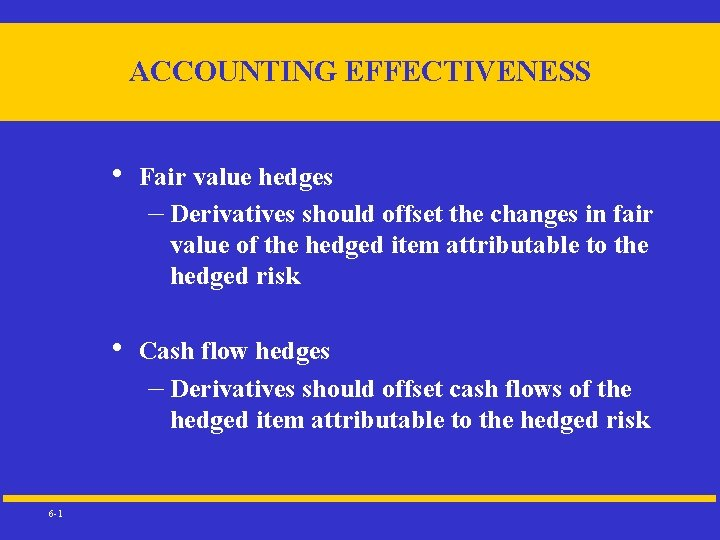ACCOUNTING EFFECTIVENESS 6 -1 • Fair value hedges – Derivatives should offset the changes in fair value of the hedged item attributable to the hedged risk • Cash flow hedges – Derivatives should offset cash flows of the hedged item attributable to the hedged risk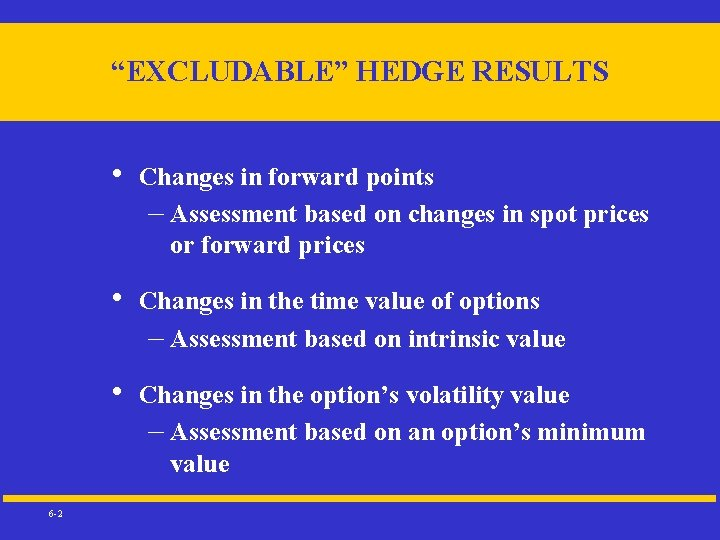“EXCLUDABLE” HEDGE RESULTS 6 -2 • Changes in forward points – Assessment based on changes in spot prices or forward prices • Changes in the time value of options – Assessment based on intrinsic value • Changes in the option’s volatility value – Assessment based on an option’s minimum value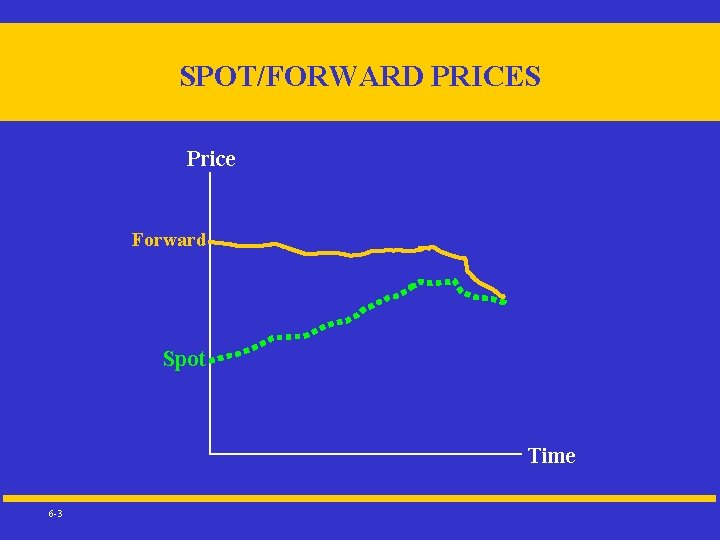SPOT/FORWARD PRICES Price Forward Spot Time 6 -3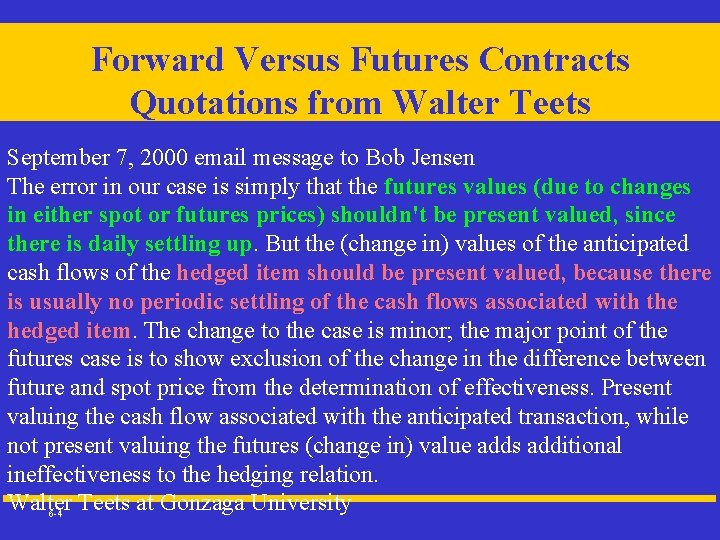Forward Versus Futures Contracts Quotations from Walter Teets September 7, 2000 email message to Bob Jensen The error in our case is simply that the futures values (due to changes in either spot or futures prices) shouldn't be present valued, since there is daily settling up. But the (change in) values of the anticipated cash flows of the hedged item should be present valued, because there is usually no periodic settling of the cash flows associated with the hedged item. The change to the case is minor; the major point of the futures case is to show exclusion of the change in the difference between future and spot price from the determination of effectiveness. Present valuing the cash flow associated with the anticipated transaction, while not present valuing the futures (change in) value adds additional ineffectiveness to the hedging relation. Walter Teets at Gonzaga University 6 -4KPMG Example 4. 2 Cumulative Dollar Offset 6 -5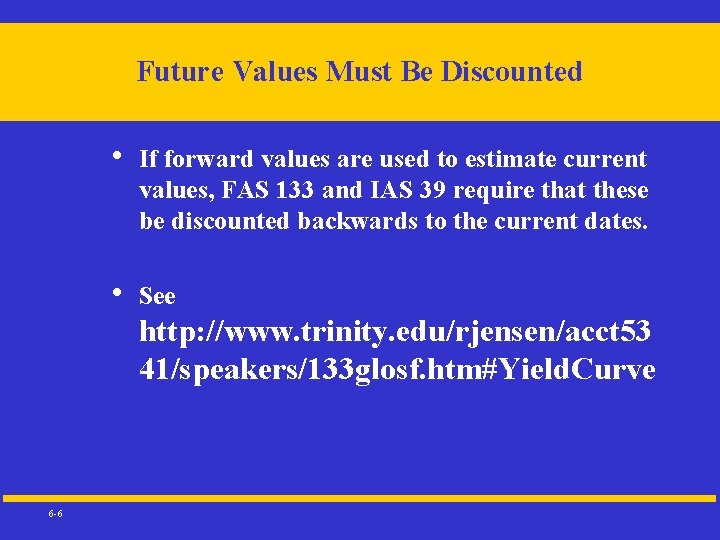Future Values Must Be Discounted • If forward values are used to estimate current values, FAS 133 and IAS 39 require that these be discounted backwards to the current dates. • See http: //www. trinity. edu/rjensen/acct 53 41/speakers/133 glosf. htm#Yield. Curve 6 -6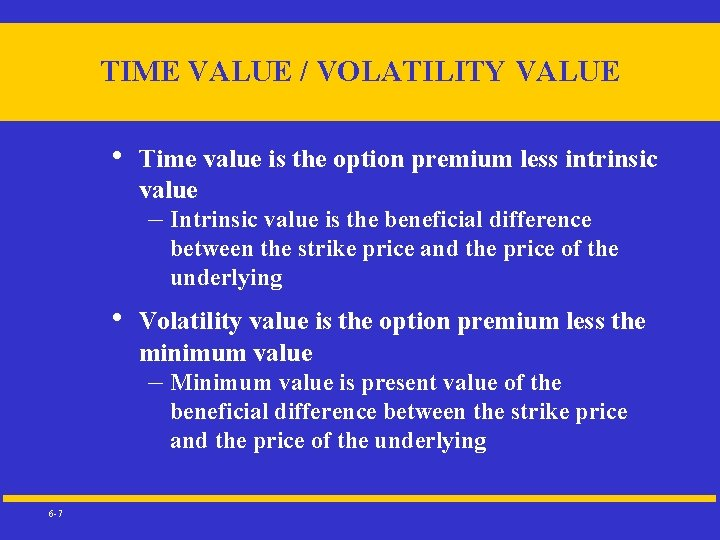TIME VALUE / VOLATILITY VALUE • Time value is the option premium less intrinsic value – Intrinsic value is the beneficial difference between the strike price and the price of the underlying • Volatility value is the option premium less the minimum value – Minimum value is present value of the beneficial difference between the strike price and the price of the underlying 6 -7FEATURES OF OPTIONS Option Value = Intrinsic Value + Time Value 6 -8 • Intrinsic Value: Difference between the strike price and the underlying price, if beneficial; otherwise zeroz • Time Value: Sensitive to time and volatility; equals zero at expiration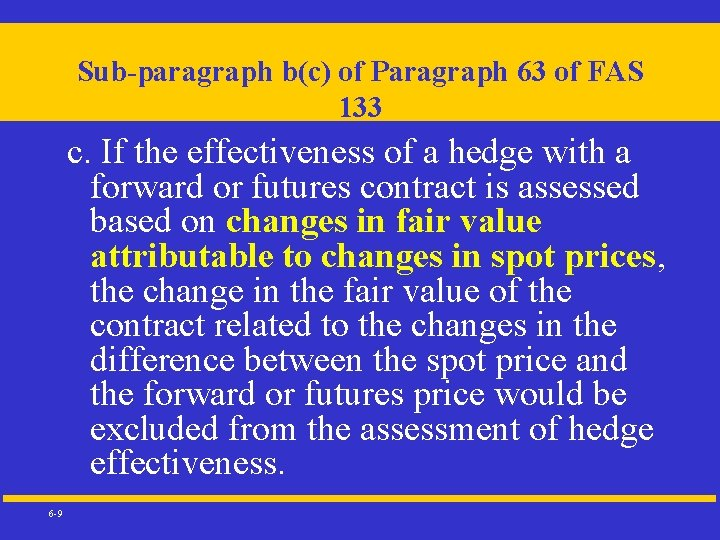Sub-paragraph b(c) of Paragraph 63 of FAS 133 c. If the effectiveness of a hedge with a forward or futures contract is assessed based on changes in fair value attributable to changes in spot prices, the change in the fair value of the contract related to the changes in the difference between the spot price and the forward or futures price would be excluded from the assessment of hedge effectiveness. 6 -9Sub-paragraph b(a) of Paragraph 63 of FAS 133 a. If the effectiveness of a hedge with an option contract is assessed based on changes in the option's intrinsic value, the change in the time value of the contract would be excluded from the assessment of hedge effectiveness. 6 -10Sub-paragraph b(b) of Paragraph 63 of FAS 133 b. If the effectiveness of a hedge with an option contract is assessed based on changes in the option's minimum value, that is, its intrinsic value plus the effect of discounting, the change in the volatility value of the contract would be excluded from the assessment of hedge effectiveness. 6 -11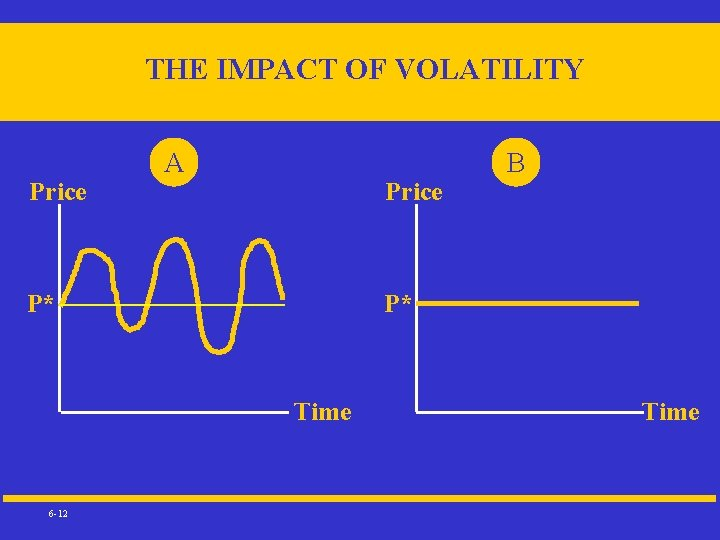THE IMPACT OF VOLATILITY Price A Price P* P* Time 6 -12 B TimeMinimum Value Option Value = Risk Free Value + Volatility Value If the underlying is the price of corn, then the minimum value of an option on corn is either zero or the current spot price of corn minus the discounted risk-free present value of the strike price. In other words if the option cannot be exercised early, discount the present value of the strike price from the date of expiration and compare it with the current spot price. If the difference is positive, this is the minimum value. It can hypothetically be the minimum value of an American option, but in an efficient market the current price of an American option will not sell below its risk free present value. 6 -13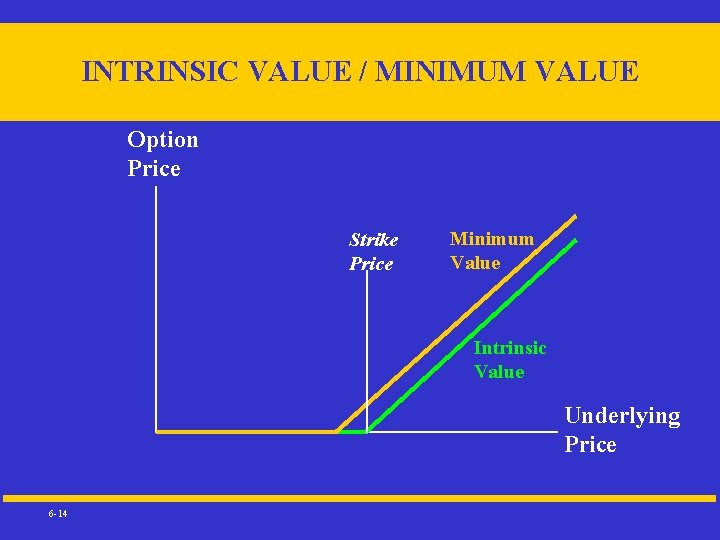INTRINSIC VALUE / MINIMUM VALUE Option Price Strike Price Minimum Value Intrinsic Value Underlying Price 6 -14Minimum (Risk Free) Versus Intrinsic Value European Call Option • • X = Exercise Price in n periods after current time P = Current Price (Underlying) of Commodity • I = P-X>0 is the intrinsic value using the current spot price if the option is in the money • M = P-[X/(1+r)n] is the minimum value at the current time • M>I if the option if the intrinsic value I is greater than zero Value of Option exceeds minimum M due to volatility value 6 -15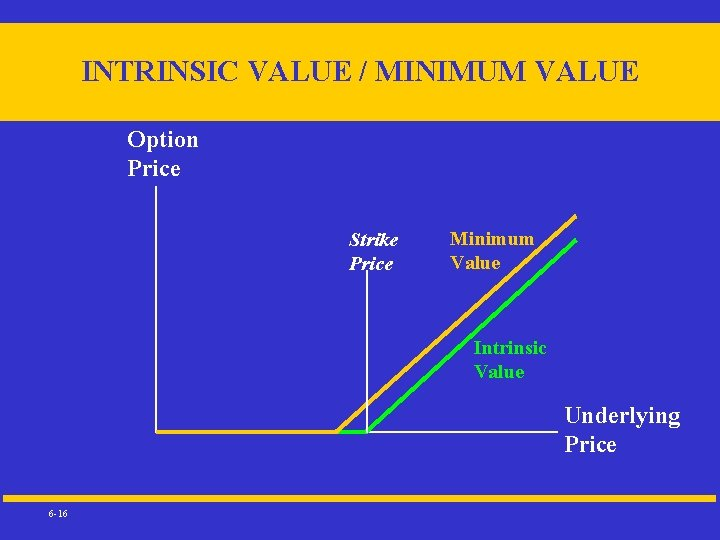INTRINSIC VALUE / MINIMUM VALUE Option Price Strike Price Minimum Value Intrinsic Value Underlying Price 6 -16Minimum Versus Intrinsic Value American Call Option • • X = Exercise Price in n periods after current time P = Current Price (Underlying) of Commodity • I = P-X>0 is the intrinsic value using the current spot price if the option is in the money • M = 0 is the minimum value since option can be exercised at any time if the option’s value is less than intrinsic value I. Value of option exceeds M and I due to volatility value 6 -17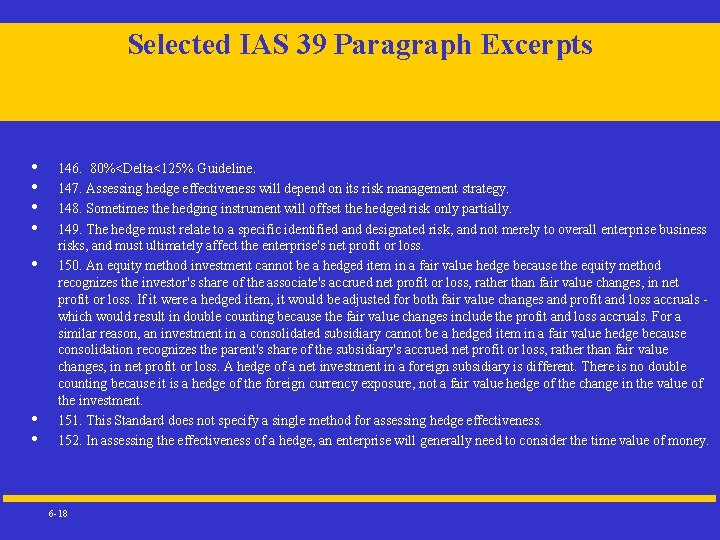Selected IAS 39 Paragraph Excerpts • • 146. 80%<Delta<125% Guideline. 147. Assessing hedge effectiveness will depend on its risk management strategy. 148. Sometimes the hedging instrument will offset the hedged risk only partially. 149. The hedge must relate to a specific identified and designated risk, and not merely to overall enterprise business risks, and must ultimately affect the enterprise's net profit or loss. 150. An equity method investment cannot be a hedged item in a fair value hedge because the equity method recognizes the investor's share of the associate's accrued net profit or loss, rather than fair value changes, in net profit or loss. If it were a hedged item, it would be adjusted for both fair value changes and profit and loss accruals - which would result in double counting because the fair value changes include the profit and loss accruals. For a similar reason, an investment in a consolidated subsidiary cannot be a hedged item in a fair value hedge because consolidation recognizes the parent's share of the subsidiary's accrued net profit or loss, rather than fair value changes, in net profit or loss. A hedge of a net investment in a foreign subsidiary is different. There is no double counting because it is a hedge of the foreign currency exposure, not a fair value hedge of the change in the value of the investment. 151. This Standard does not specify a single method for assessing hedge effectiveness. 152. In assessing the effectiveness of a hedge, an enterprise will generally need to consider the time value of money. 6 -18FAS Effectiveness Testing --- http: //www. qrm. com/products/mb/Rmbupdate. htm • • • Dollar Offset (DO) calculates the ratio of dollar change in profit/loss for hedge and hedged item Relative Dollar Offset (RDO) calculates the ratio of dollar change in net position to the initial MTM value of hedged item Variability Reduction Measure (Var. RM) calculates the ratio of the squared dollar changes in net position to the squared dollar changes in hedged item Ordinary Least Square (OLS) measures the linear relationship between the dollar changes in hedged item and hedge. OLS calculates the coefficient of determination (R 2) and the slope coefficient (ß) for effectiveness measure and accounts for the historical performance Least Absolute Deviation (LAD) is similar to OLS, but employs median regression analysis to calculate R 2 and ß. 6 -19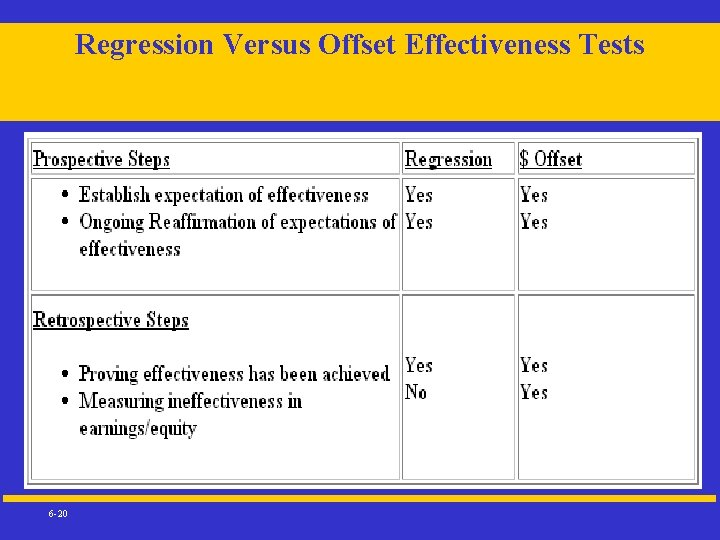Regression Versus Offset Effectiveness Tests 6 -20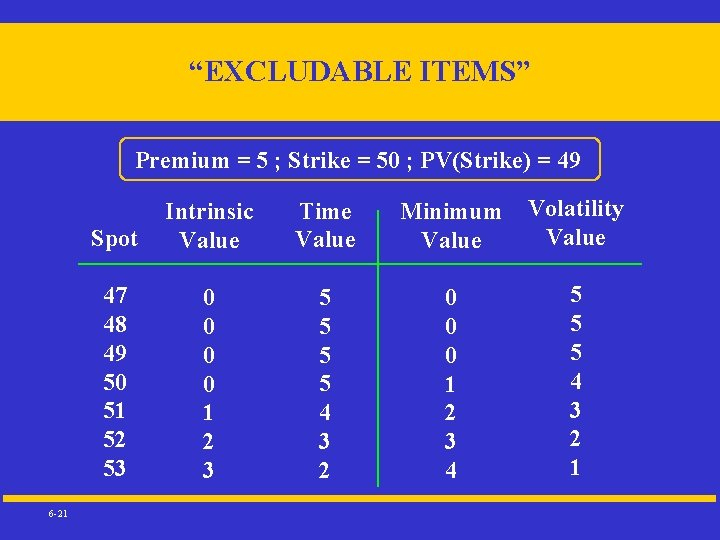“EXCLUDABLE ITEMS” Premium = 5 ; Strike = 50 ; PV(Strike) = 49 6 -21 Spot Intrinsic Value Time Value Minimum Value Volatility Value 47 48 49 50 51 52 53 0 0 1 2 3 5 5 4 3 2 0 0 0 1 2 3 4 5 5 5 4 3 2 1LONG OPTION HEDGES • Fair value hedges – Mark-to-market of the option will generally be smaller than exposure’s contribution to earnings • Cash flow hedges – Changes in intrinsic values of options go to – other comprehensive income to the extent effective* Remaining changes in option prices goes to current income * Bounded by the magnitude of the exposures’ price changes 6 -22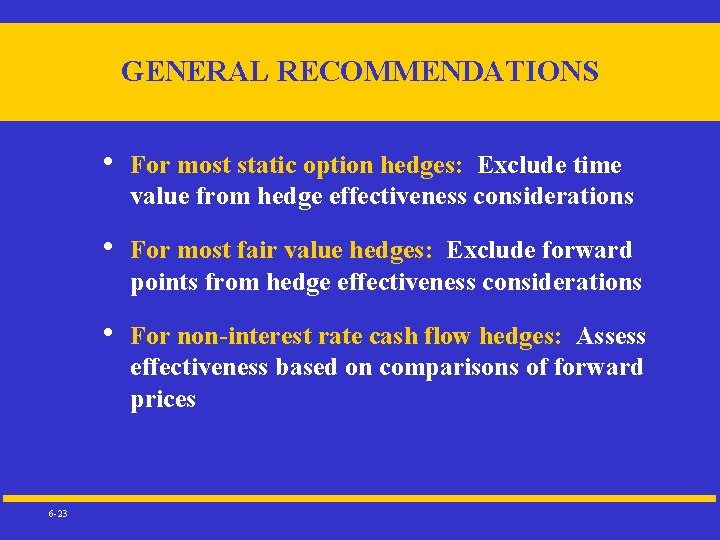GENERAL RECOMMENDATIONS 6 -23 • For most static option hedges: Exclude time value from hedge effectiveness considerations • For most fair value hedges: Exclude forward points from hedge effectiveness considerations • For non-interest rate cash flow hedges: Assess effectiveness based on comparisons of forward prices“THE RISK BEING HEDGED” • For non-interest rate exposures – Entities must identify their firm-specific exposures as hedged items – Differences between firm-specific prices and hedging instruments’ underlying variables will foster income volatility – Pre-qualifying hedge effectiveness documentation is required for all crosshedges 6 -24Sub-paragraph b(c) of Paragraph 63 of FAS 133 c. If the effectiveness of a hedge with a forward or futures contract is assessed based on changes in fair value attributable to changes in spot prices, the change in the fair value of the contract related to the changes in the difference between the spot price and the forward or futures price would be excluded from the assessment of hedge effectiveness. 6 -25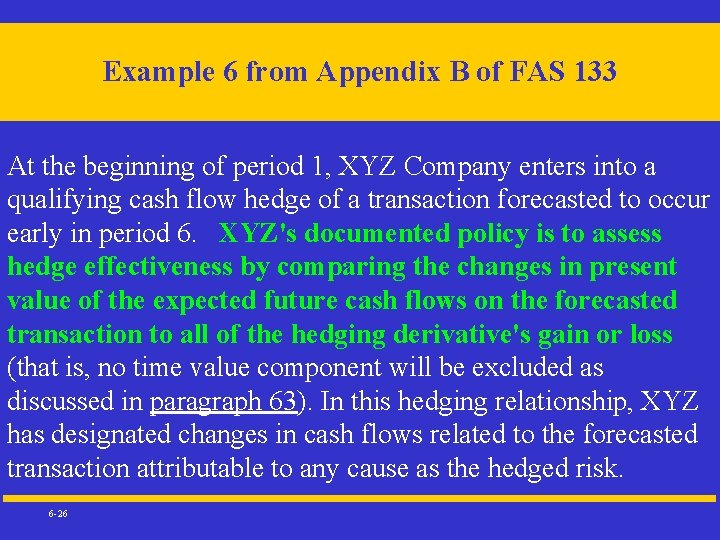Example 6 from Appendix B of FAS 133 At the beginning of period 1, XYZ Company enters into a qualifying cash flow hedge of a transaction forecasted to occur early in period 6. XYZ's documented policy is to assess hedge effectiveness by comparing the changes in present value of the expected future cash flows on the forecasted transaction to all of the hedging derivative's gain or loss (that is, no time value component will be excluded as discussed in paragraph 63). In this hedging relationship, XYZ has designated changes in cash flows related to the forecasted transaction attributable to any cause as the hedged risk. 6 -26Example 6 in Appendix B of FAS 133 From File 133 ex 06 a. xls 6 -27“HEDGEABLE” INTEREST RATE RISKS • • 6 -28 Overall fair value effects Fair value changes due to changes in a benchmark interest rate (i. e. , the risk-free rate or the LIBOR-based swap rate) Fair value changes due to interest rate effects associated with credit quality and/or rating changes Fair value changes due to foreign exchange rate changesFAIR VALUE INTEREST RATE HEDGES 6 -29 • The benchmark interest rate will likely be the predominant selection for the hedged item • The shortcut method is only applicable for interest rate swaps if all the criteria of ¶’s 68 and 69 are met • Fair value hedges that don’t qualify for shortcut may have considerable unintended income effects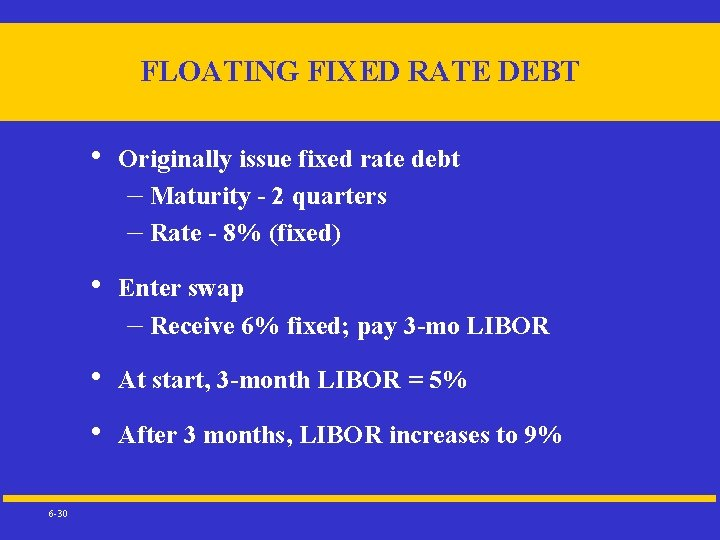FLOATING FIXED RATE DEBT 6 -30 • Originally issue fixed rate debt – Maturity - 2 quarters – Rate - 8% (fixed) • Enter swap – Receive 6% fixed; pay 3 -mo LIBOR • At start, 3 -month LIBOR = 5% • After 3 months, LIBOR increases to 9%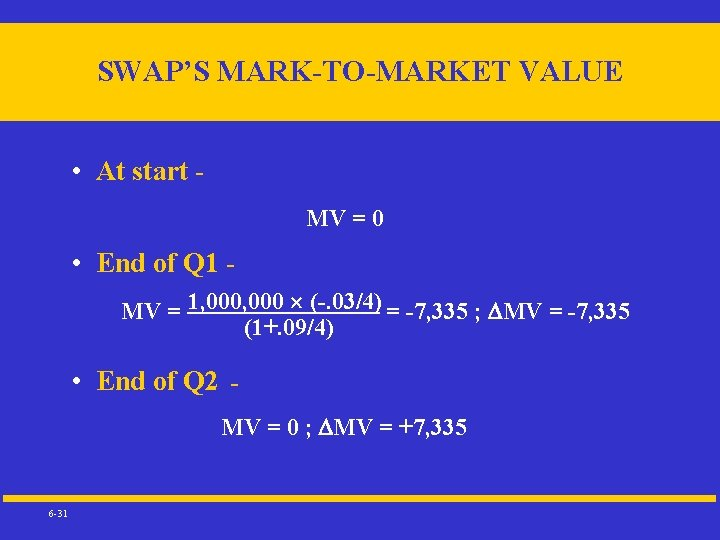SWAP’S MARK-TO-MARKET VALUE • At start MV = 0 • End of Q 1 MV = 1, 000 (-. 03/4) = -7, 335 ; MV = -7, 335 (1+. 09/4) • End of Q 2 MV = 0 ; MV = +7, 335 6 -31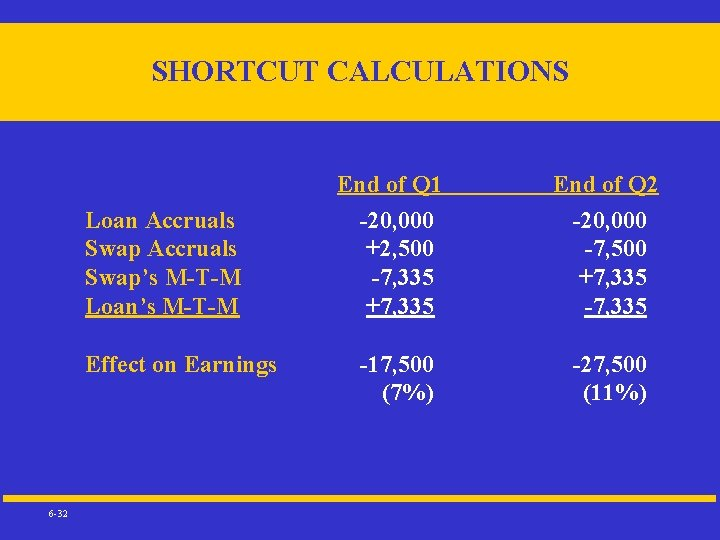SHORTCUT CALCULATIONS Loan Accruals Swap’s M-T-M Loan’s M-T-M Effect on Earnings 6 -32 End of Q 1 -20, 000 +2, 500 -7, 335 +7, 335 End of Q 2 -20, 000 -7, 500 +7, 335 -17, 500 (7%) -27, 500 (11%)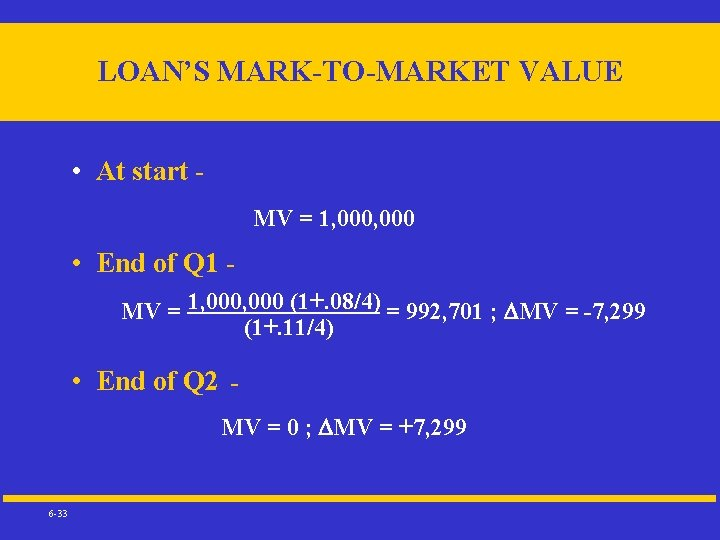LOAN’S MARK-TO-MARKET VALUE • At start MV = 1, 000 • End of Q 1 MV = 1, 000 (1+. 08/4) = 992, 701 ; MV = -7, 299 (1+. 11/4) • End of Q 2 MV = 0 ; MV = +7, 299 6 -33NON-SHORTCUT CALCULATIONS Loan Accruals Swap’s M-T-M Loan’s M-T-M Effect on Earnings 6 -34 End of Q 1 -20, 000 +2, 500 -7, 335 +7, 299 End of Q 2 -20, 000 -7, 500 +7, 335 -7, 299 -17, 536 (7. 01%) -27, 464 (10. 99%)PERSPECTIVES ON INEFFECTIVENSS Impact of 50 basis point fall in interest rates on \$1 million par bonds; semi-annual compounding 6 -35 6%-Coupon 9%-Coupon Difference 1 -Yr \$4, 801 \$4, 698 \$103 (4 b. p. ) 5 -Yr \$21, 600 \$20, 027 \$1, 573 (63 b. p. ) 10 -Yr \$38, 068 \$33, 236 \$4, 832 (193 b. p. )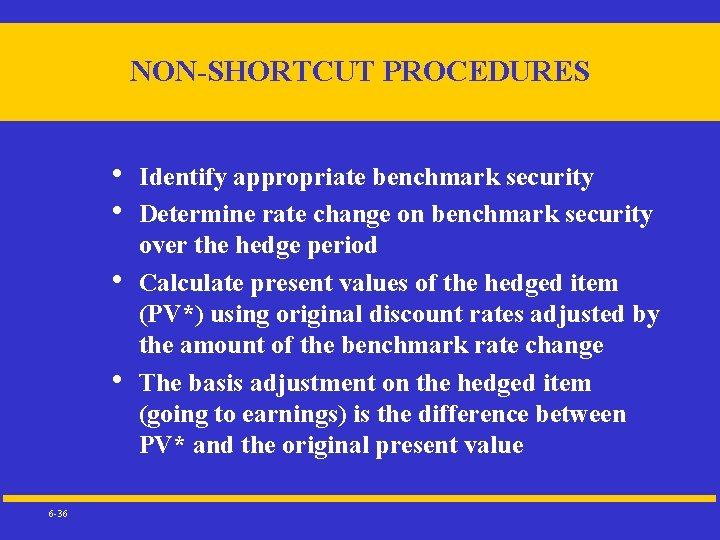NON-SHORTCUT PROCEDURES • • 6 -36 Identify appropriate benchmark security Determine rate change on benchmark security over the hedge period Calculate present values of the hedged item (PV*) using original discount rates adjusted by the amount of the benchmark rate change The basis adjustment on the hedged item (going to earnings) is the difference between PV* and the original present value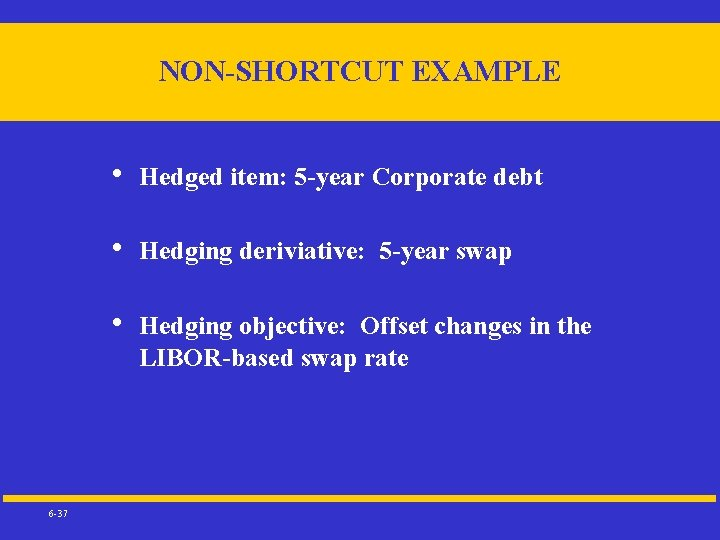NON-SHORTCUT EXAMPLE 6 -37 • Hedged item: 5 -year Corporate debt • Hedging deriviative: 5 -year swap • Hedging objective: Offset changes in the LIBOR-based swap rate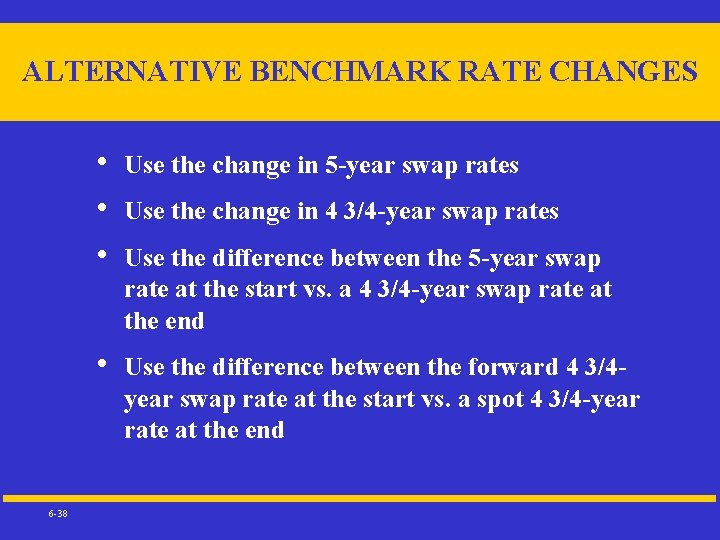ALTERNATIVE BENCHMARK RATE CHANGES 6 -38 • • • Use the change in 5 -year swap rates • Use the difference between the forward 4 3/4 year swap rate at the start vs. a spot 4 3/4 -year rate at the end Use the change in 4 3/4 -year swap rates Use the difference between the 5 -year swap rate at the start vs. a 4 3/4 -year swap rate at the end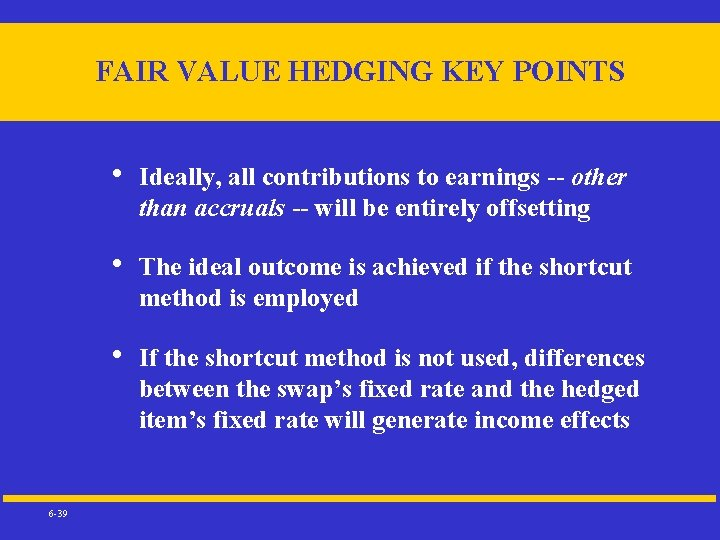FAIR VALUE HEDGING KEY POINTS 6 -39 • Ideally, all contributions to earnings -- other than accruals -- will be entirely offsetting • The ideal outcome is achieved if the shortcut method is employed • If the shortcut method is not used, differences between the swap’s fixed rate and the hedged item’s fixed rate will generate income effects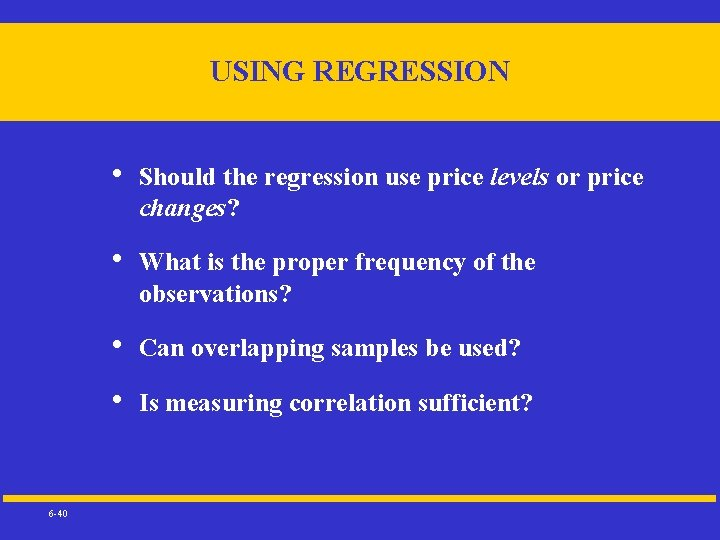USING REGRESSION 6 -40 • Should the regression use price levels or price changes? • What is the proper frequency of the observations? • Can overlapping samples be used? • Is measuring correlation sufficient?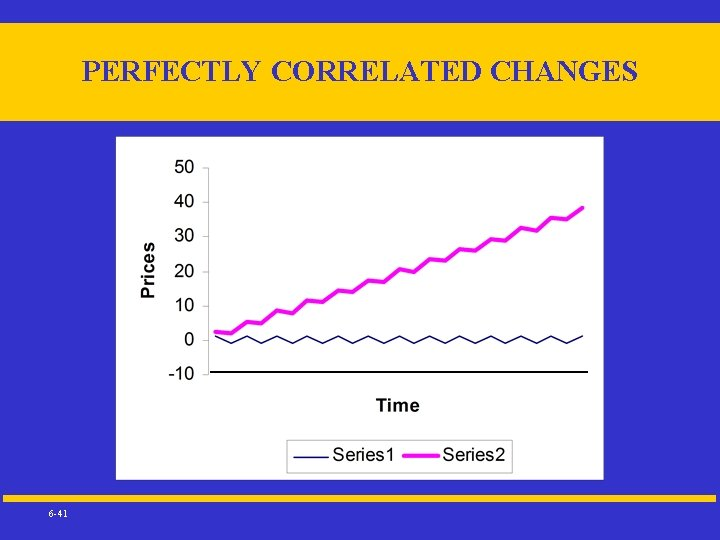PERFECTLY CORRELATED CHANGES 6 -41AN ALTERNATIVE TO REGRESSION • • 6 -42 Given time series of price changes for both the derivative and the hedged item, generate a frequency distribution for the combined results Assign a threshold value and a level of confidence for “high effectiveness” (e. g. , the combined results must be smaller than 2% of the initial value of the hedged item in 95% of the observations)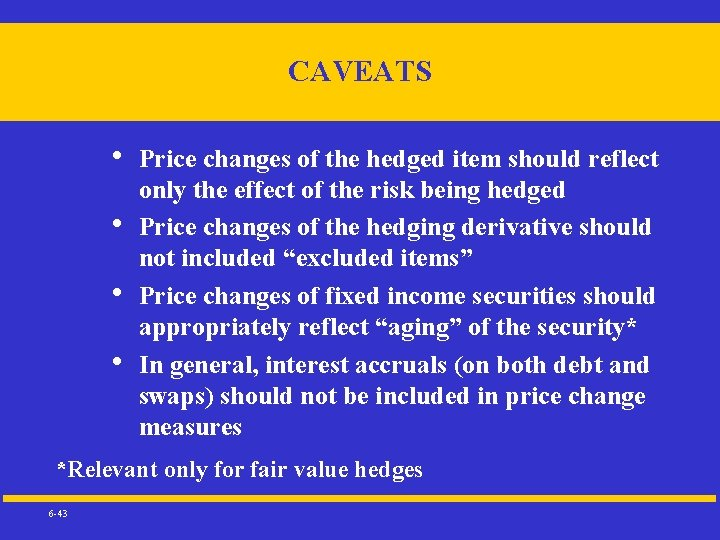CAVEATS • • Price changes of the hedged item should reflect only the effect of the risk being hedged Price changes of the hedging derivative should not included “excluded items” Price changes of fixed income securities should appropriately reflect “aging” of the security* In general, interest accruals (on both debt and swaps) should not be included in price change measures *Relevant only for fair value hedges 6 -43Shortcut Method conditions applicable to both fair value hedges and cash flow hedges • The notional amount of the swap matches the principal amount of the interest-bearing asset or liability being hedged. If the hedging instrument is solely an interest rate swap, the fair value of the swap at its inception is zero. There are other applicable conditions if the instrument is a compound derivative composed of an interest rate swap and an option. The formula for computing net settlements under the interest rate swap is the same for each net settlement. (That is, the fixed rate is the same throughout the term, and the variable rate is based on the same index and includes the same constant adjustment or no adjustment. ) The interest-bearing asset or liability is not prepayable, unless the asset or liability is prepayable solely due to an embedded option and the hedging instrument is a compound derivative composed of an interest rate swap and an option. • • • 6 -44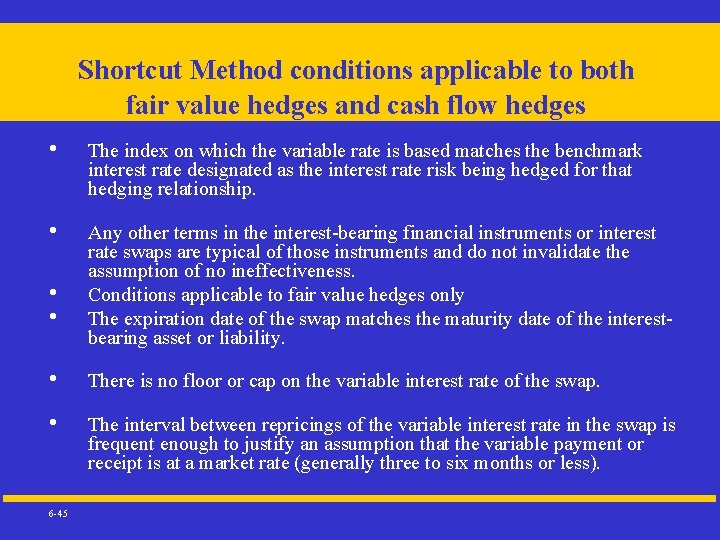Shortcut Method conditions applicable to both fair value hedges and cash flow hedges • • • 6 -45 The index on which the variable rate is based matches the benchmark interest rate designated as the interest rate risk being hedged for that hedging relationship. Any other terms in the interest-bearing financial instruments or interest rate swaps are typical of those instruments and do not invalidate the assumption of no ineffectiveness. Conditions applicable to fair value hedges only The expiration date of the swap matches the maturity date of the interestbearing asset or liability. There is no floor or cap on the variable interest rate of the swap. The interval between repricings of the variable interest rate in the swap is frequent enough to justify an assumption that the variable payment or receipt is at a market rate (generally three to six months or less).Shortcut Method conditions applicable to both fair value hedges and cash flow hedges • • • 6 -46 The index on which the variable rate is based matches the benchmark interest rate designated as the interest rate risk being hedged for that hedging relationship. Any other terms in the interest-bearing financial instruments or interest rate swaps are typical of those instruments and do not invalidate the assumption of no ineffectiveness. Conditions applicable to fair value hedges only The expiration date of the swap matches the maturity date of the interestbearing asset or liability. There is no floor or cap on the variable interest rate of the swap. The interval between repricings of the variable interest rate in the swap is frequent enough to justify an assumption that the variable payment or receipt is at a market rate (generally three to six months or less).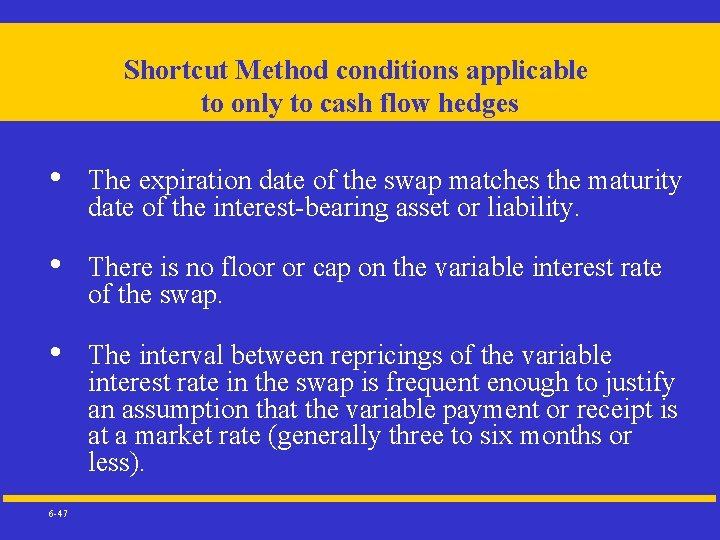Shortcut Method conditions applicable to only to cash flow hedges • • • 6 -47 The expiration date of the swap matches the maturity date of the interest-bearing asset or liability. There is no floor or cap on the variable interest rate of the swap. The interval between repricings of the variable interest rate in the swap is frequent enough to justify an assumption that the variable payment or receipt is at a market rate (generally three to six months or less).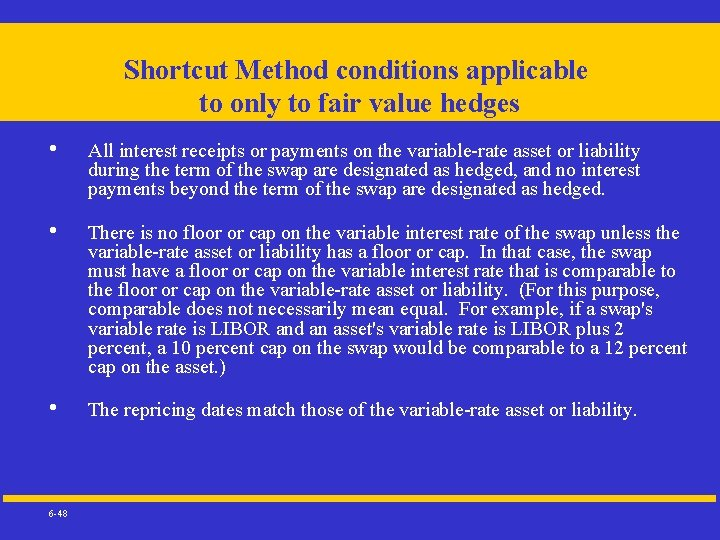Shortcut Method conditions applicable to only to fair value hedges • • • 6 -48 All interest receipts or payments on the variable-rate asset or liability during the term of the swap are designated as hedged, and no interest payments beyond the term of the swap are designated as hedged. There is no floor or cap on the variable interest rate of the swap unless the variable-rate asset or liability has a floor or cap. In that case, the swap must have a floor or cap on the variable interest rate that is comparable to the floor or cap on the variable-rate asset or liability. (For this purpose, comparable does not necessarily mean equal. For example, if a swap's variable rate is LIBOR and an asset's variable rate is LIBOR plus 2 percent, a 10 percent cap on the swap would be comparable to a 12 percent cap on the asset. ) The repricing dates match those of the variable-rate asset or liability.